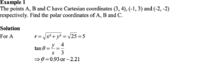# Speaking of tan graphs..I am confused about polar curves and the theta derived from inverse tan.

#### Sonal7

##### Full Member
I am learning polar curves at the moment. I am wondering about this example. I understand the first value0.93 has come from, but I don't understand where the second figure has come from -2.21. Sorry to be a dumbo. I know CAST diagram but I am unclear on what's going on here. I know about the graph of TanI am not sure why tossed between the two and not go for more positive value $$\displaystyle theta + pi$$

#### Attachments

• 70 KB Views: 3
• 48.2 KB Views: 3

#### Harry_the_cat

##### Senior Member
OK -2.21 = 0.93 - pi (this is to get the angle in the third quadrant using CAST)

Yes, 0.93 + pi would also be a solution to the equation.

However, it is conventional to give what is called the Principal Argument. That is a value between -pi and pi. -pi<Principal Argument <= pi.

Note that 0.93 lies within this range as does 0.93 - pi. But 0.93+pi doesn't.

•Sonal7

#### Otis

##### Senior Member
… I don't understand where the [-2.21] has come from …
Hi Sonal. I don't understand why they listed -2.21, either. You may ignore it.

A calculator outputs one angle for arctangent (because the domain has to be restricted to one period, for arctan to be a function), and the angle output is always in Q1 or Q4. We then adjust the output, depending on the quadrant where the terminal ray of the angle is.

In your exercise, the given point is in Q1, and the arctan value output is for the angle whose terminal ray lies in Q1, so no adjustment to the output 0.93 is needed.

Do you have a textbook that defines the inverse trig functions?#### Sonal7

##### Full Member
Yes i am sure every A level maths textbook should have a chapter. I shall look it up.

#### Sonal7

##### Full Member
OK -2.21 = 0.93 - pi (this is to get the angle in the third quadrant using CAST)

Yes, 0.93 + pi would also be a solution to the equation.

However, it is conventional to give what is called the Principal Argument. That is a value between -pi and pi. -pi<Principal Argument <= pi.

Note that 0.93 lies within this range as does 0.93 - pi. But 0.93+pi doesn't.
Yes we did cover this in complex numbers. Its the angle made with the positive x axis and the line. I remember this now. Sorry to ask such a question. I need to buy a rubber duck and speak to it for 10 minutes. Thanks this is helpful. I suppose its convention.

#### Harry_the_cat

##### Senior Member
Yeah I just reread the problem you posted. Did the next line in the solution (which has been cropped) go on to say that for A, which is in the first quadrant, then the angle is 0.93 and -2.21 is invalid?

#### Otis

##### Senior Member
•Sonal7

#### Sonal7

##### Full Member
Just looking at polar co-ordinates as they are interesting. Thank you for the link. Might glean now.

#### Sonal7

##### Full Member
Yeah I just reread the problem you posted. Did the next line in the solution (which has been cropped) go on to say that for A, which is in the first quadrant, then the angle is 0.93 and -2.21 is invalid?
Yes thats what is implied.

#### Attachments

• 60.5 KB Views: 3
•Harry_the_cat

#### Sonal7

##### Full Member
Here are two relevant links, for those interested.

CartesianPolar (conversions)

Inverse trig functions (for sine, cosine and tangent)I think it may some thing to do with the inverse trig function. $$\displaystyle -\pi\leq\theta\leq\pi$$. I am not sure but its a thought.#### Harry_the_cat

##### Senior Member
I think it may some thing to do with the inverse trig function. $$\displaystyle -\pi\leq\theta\leq\pi$$. I am not sure but its a thought.Your first <= should be just<.

#### Sonal7

##### Full Member
Okay. That makes sense.